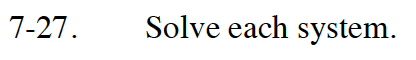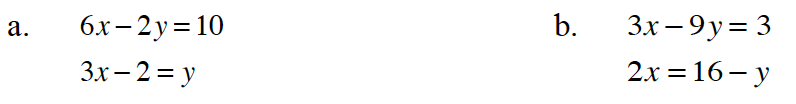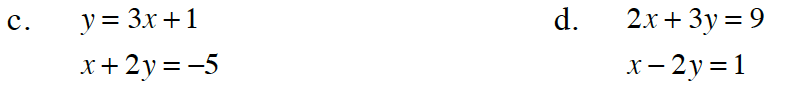Home > CC4 > Chapter 7 > Lesson 7.1.2 > Problem7-27

7-27.

Solve each system. Homework Help ✎

1. 6x − 2y = 10
3x − 2 = y

2. 3x − 9y = 3
2x = 16 − y

3. y = 3x + 1
x + 2y = −5

4. 2x + 3y = 9
x − 2y = 1Read Math Notes box in Lesson 6.2.3 on the substitution method.There is no solution.

Solve the lower equation for y, and then substitute the expression for y into the top equation and solve.Substitute 3x + 1 for y in the second equation.

(−1, −2)

Multiply the entire second equation by −2

Add the equations together.

(3, 1)